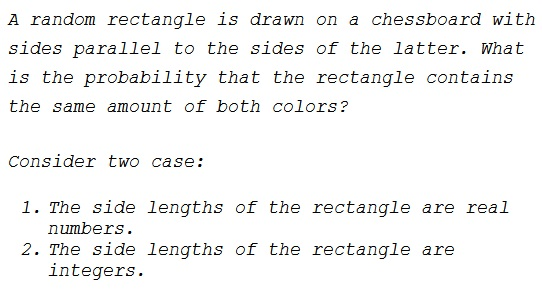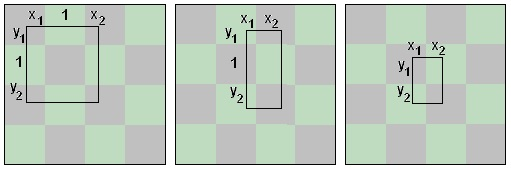# Probability of Equal Areas on a Chessboard

### Problem### Claim

A rectangle with sides parallel to the sides of a chessboard covers equal areas of both colors if and only if one of the two conditions holds:

1. One of the sides of the rectangle is an even multiple of the side of a chessboard square. The dimension of the other side is irrelevant.

2. The center of the rectangle lies on a grid line.

### Solution

For simplicity, let's call any segment double a square's side in length even.

The sufficiency (the "if" part) is pretty obvious. If, say, the vertical dimension of the rectangle is double a square's side, then in every vertical cross-section of the rectangle segments of different colors add up to the same lengths. Also, if the center of the rectangle lies on a grid line, then by symmetry the statement is also true.

Observe that every rectangle can be split into at most three: one with an even horizontal dimension, one with an even vertical dimension, and one with both dimensions less than 2.

The problem of showing the necessity, i.e. the "only if" part, is thus reduced to showing that in a rectangle with sides less than $2$ there is more of one color than the other, provided the center of the rectangle does not lie on a grid line. There are three prototypical possibilities:In all cases,

$0 \lt x_{1}, x_{2} \lt 1$ and $0 \lt y_{1}, y_{2} \lt 1.$

Also,

$0 \lt x_{1} + x_{2} \lt 1$

in the first case, and

$0 \lt y_{1} + y_{2} \lt 1$

in cases 1 and 2.

If $A_{1}$ and $A_{2}$ are total areas of the regions of the light and dark colors inside the rectangle, then, in the first case we get

\begin{align} A_{1} &= x_{1} + x_{2} + y_{1} + y_{2},\\ A_{2} &= (x_{1} + x_{2})(y_{1} + y_{2}) + 1. \end{align}

The difference of the two is

$A_{1} - A_{2} = -(x_{1} + x_{2} - 1)(y_{1} + y_{2} - 1),$

which is never 0.

In the second case we get

\begin{align} A_{1} &= x_{1}y_{1} + x_{2} + x_{1}y_{2} = x_{1}(y_{1} + y_{2}) + x_{2},\\ A_{2} &= x_{2}y_{1} + x_{1} + x_{2}y_{2} = x_{2}(y_{1} + y_{2}) + x_{1}. \end{align}

The difference of the two is

$A_{1} - A_{2} = (x_{1} - x_{2})(y_{1} + y_{2} - 1),$

which may be $0$ iff $x_{1} = x_{2},$ i.e, in the excluded case where the center of the rectangle lies on a grid line. The last case is treated similarly.

Due to the fact that the probability of a random rectangle having the center on a grid line is zero, the problem has an unexpected solution: for a rectangle with an even side, the probability of covering equal white and black areas is $1;$ for the less fortunate rectangles, the probability is $0.$

As having an integer, let alone an even, side has the probability of zero, $0$ is the answer to the first question.

For the second question, the sides of the rectangle may be independently odd or even. There are several interpretations.

One answer is that in three out of four possibilities at least one side is even, making the probability of having equal areas $\displaystyle \frac{3}{4}.$

However, this is a simplified treatment of the problem. The availability of even and odd side lengths may depend on the distance of a selected corner to the sides of the chessboard.

On an $8\times 8$ board, integer rectangles may be of size $M\times N,$ where $1\le M,N\le 7.$ Thus, for $M=2,4,6,$ there are $7$ rectangles with at least one side even, $21,$ in all. For each of $M=1,3,5,7,$ there are $3$ rectangles with at least one side even, $12,$ in all. So the total number of integer rectangular sizes that cover equal white and black areas is $21+12=33.$ This is out of the total of $7\times 7=49$ possible sizes, giving the probability of $\displaystyle \frac{33}{49}.$

If we count the frequencies of various rectangle sizes, the answer changes again. Below the sizes $M\times N$ are tabulated separately depending on odd/even combination of side lengths:

$\begin{array}{r|r|r|r|r|} M/N&1&3&5&7\\ \hline 1&64&48&32&16\\ \hline 3&48&36&24&12\\ \hline 5&32&24&16&8\\ \hline 7&16&12&8&4\\ \hline \hline &Total:&&400& \end{array} \qquad\qquad \begin{array}{r|r|r|r|r|} M/N&1&3&5&7\\ \hline 2&56&42&28&14\\ \hline 4&40&30&20&10\\ \hline 6&24&18&12&6\\ \hline 8&8&6&4&2\\ \hline \hline &Total:&320\times 2&=640&\\ \end{array}$

$\begin{array}{r|r|r|r|r|} M/N&2&4&6&8\\ \hline 2&49&35&21&7\\ \hline 4&35&25&15&5\\ \hline 6&21&15&9&3\\ \hline 8&7&5&3&1\\ \hline \hline &Total:&&256&\\ \end{array}$

Thus an interger rectangle covers equal areas of black and white in $896=640+256$ out of $400+896=1296$ cases, i.e., with the probability of $\displaystyle \frac{896}{1296}=\frac{56}{81}.$

### Acknowledgment

This is a remake of an old page with a focus on the probabilistic aspect of the problem, with a proof by Nathan Bowler. I am indebted to Nathan for pointing out an omission in the initial variant of the statement. Nathan continues the discussion elsewhere.

The tabulated calculations above are due to Thamizh Kudimagan.

### Geometric Probability